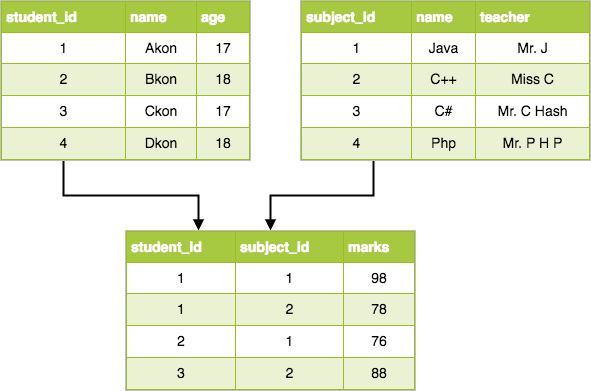# Types of DBMS: Relational

Relational data model is the primary data model, which is used widely around the world for data storage and processing. This model is simple and it has all the properties and capabilities required to process data with storage efficiency.

### Concepts

Tables − In relational data model, relations are saved in the format of Tables. This format stores the relation among entities. A table has rows and columns, where rows represents records and columns represent the attributes.

Tuple − A single row of a table, which contains a single record for that relation is called a tuple.

Relation instance − A finite set of tuples in the relational database system represents relation instance. Relation instances do not have duplicate tuples.

Relation schema − A relation schema describes the relation name (table name), attributes, and their names.

Relation key − Each row has one or more attributes, known as relation key, which can identify the row in the relation (table) uniquely.

Attribute domain − Every attribute has some pre-defined value scope, known as attribute domain.

### Constraints

Every relation has some conditions that must hold for it to be a valid relation. These conditions are called Relational Integrity Constraints. There are three main integrity constraints −

• Key constraints
• Domain constraints
• Referential integrity constraints

### Key Constraints

There must be at least one minimal subset of attributes in the relation, which can identify a tuple uniquely. This minimal subset of attributes is called key for that relation. If there are more than one such minimal subsets, these are called candidate keys.

Key constraints force that:

• in a relation with a key attribute, no two tuples can have identical values for key attributes.
• a key attribute can not have NULL values.

Key constraints are also referred to as Entity Constraints.

### Domain Constraints

Attributes have specific values in real-world scenario. For example, age can only be a positive integer. The same constraints have been tried to employ on the attributes of a relation. Every attribute is bound to have a specific range of values. For example, age cannot be less than zero and telephone numbers cannot contain a digit outside 0-9.

### Referential integrity Constraints

Referential integrity constraints work on the concept of Foreign Keys. A foreign key is a key attribute of a relation that can be referred in other relation.

Referential integrity constraint states that if a relation refers to a key attribute of a different or same relation, then that key element must exist.## One thought on “Types of DBMS: Relational”

error: Content is protected !!